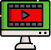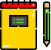VideosNotes

### Calculations Involving Multiplication And Division - 1

Calculations Involving Multiplic...
In this lesson, learners will be introduced to calculations involving multiplication and division of numbers using logarithm.
Views: N/A
Video Duration: 9:28 (Part 1 of 5)